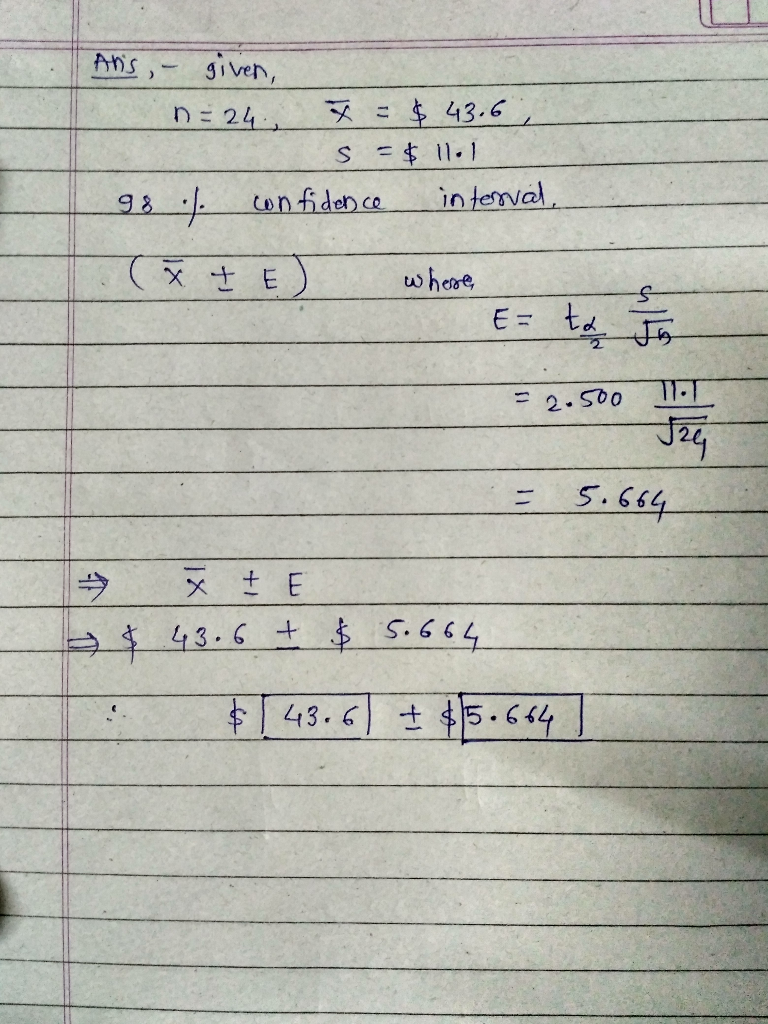Question

# In a survey, 24 people were asked how much they spent on their child's last birthday...

In a survey, 24 people were asked how much they spent on their child's last birthday gift. The results were roughly bell-shaped with a mean of \$43.6 and standard deviation of \$11.1. Estimate how much a typical parent would spend on their child's birthday gift (use a 98% confidence level). Give your answers to 3 decimal places.

\$ ___ ± \$ ___#### Earn Coins

Coins can be redeemed for fabulous gifts.

Similar Homework Help Questions
• ### In a survey, 21 people were asked how much they spent on their child's last birthday...

In a survey, 21 people were asked how much they spent on their child's last birthday gift. The results were roughly bell-shaped with a mean of \$36.4 and standard deviation of \$13.4. Estimate how much a typical parent would spend on their child's birthday gift (use a 80% confidence level). Give your answers to 3 decimal places. Express your answer in the format of x̅ ± E.

• ### In a survey, 25 people were asked how much they spent on their child's last birthday...

In a survey, 25 people were asked how much they spent on their child's last birthday gift. The results were roughly bell-shaped with a mean of \$49 and standard deviation of \$11. Estimate how much a typical parent would spend on their child's birthday gift (use a 98% confidence level). Give your answers to one decimal place. Provide the point estimate and margin or error.

• ### In a survey, 22 people were asked how much they spent on their child's last birthday...

In a survey, 22 people were asked how much they spent on their child's last birthday gift. The results were roughly bell-shaped with a mean of \$46 and standard deviation of \$12. Estimate how much a typical parent would spend on their child's birthday gift (use a 98% confidence level). Give your answers to one decimal place. Provide the point estimate and margin or error. ±± Do you add ( ) when answering?

• ### In a survey, 13 people were asked how much they spent on their child's last birthday...

In a survey, 13 people were asked how much they spent on their child's last birthday gift. The results were roughly bell-shaped with a mean of \$39 and standard deviation of \$18. Estimate how much a typical parent would spend on their child's birthday gift (use a 95% confidence level). Give your answers to one decimal place. Provide the point estimate and margin or error.

• ### In a survey, 25 people were asked how much they spent on their child's last birthday...

In a survey, 25 people were asked how much they spent on their child's last birthday gift. The results were roughly bell-shaped with a mean of \$36 and standard deviation of \$16. Estimate how much a typical parent would spend on their child's birthday gift (use a 95% confidence level). Give your answers to one decimal place. Provide the point estimate and margin or error.

• ### In a survey, 16 people were asked how much they spent on their child's last birthday...

In a survey, 16 people were asked how much they spent on their child's last birthday gift. The results were roughly bell-shaped with a mean of \$38 and standard deviation of \$13. Estimate how much a typical parent would spend on their child's birthday gift (use a 99% confidence level). Give your answers to one decimal place. Provide the point estimate and margin or error. ±±

• ### In a survey, 15 people were asked how much they spent on their child's last birthday gift. The results were roughly bell...

In a survey, 15 people were asked how much they spent on their child's last birthday gift. The results were roughly bell-shaped with a mean of \$42 and standard deviation of \$5. Estimate how much a typical parent would spend on their child's birthday gift (use a 90% confidence level).Give your answers to one decimal place. Provide the point estimate and margin or error.Do you add ( ) when answering?

• ### In a survey, 39 people were asked how much they spent on their child's last birthday...

In a survey, 39 people were asked how much they spent on their child's last birthday gift. The results were roughly bell-shaped with a mean of \$40 and standard deviation of \$4. Calculate, state, and interpret a 95% confidence interval to estimate the mean amount of money parents spend on their child's birthday gift. Round to the nearest 100th where necessary.

• ### In a survey, 30 people were asked how much they spent on their child's last birthday...

In a survey, 30 people were asked how much they spent on their child's last birthday gift. The results were roughly bell-shaped with a mean of \$37 and standard deviation of \$13. Construct a confidence interval at a 98% confidence level. Give your answers to one decimal place.

• ### In a survey, 15 people were asked how much they spent on their child's last birthday...

In a survey, 15 people were asked how much they spent on their child's last birthday gift. The results were roughly bell-shaped with a mean of \$40 and standard deviation of \$7. Find the margin of error at a 95% confidence level.